# 7.1.   二叉树¶

「二叉树 Binary Tree」是一种非线性数据结构，代表着祖先与后代之间的派生关系，体现着“一分为二”的分治逻辑。类似于链表，二叉树也是以结点为单位存储的，结点包含「值」和两个「指针」。

/* 二叉树结点类 */
class TreeNode {
int val;         // 结点值
TreeNode left;   // 左子结点指针
TreeNode right;  // 右子结点指针
TreeNode(int x) { val = x; }
}

/* 二叉树结点结构体 */
struct TreeNode {
int val;          // 结点值
TreeNode *left;   // 左子结点指针
TreeNode *right;  // 右子结点指针
TreeNode(int x) : val(x), left(nullptr), right(nullptr) {}
};

""" 二叉树结点类 """
class TreeNode:
def __init__(self, val: int):
self.val: int = val                   # 结点值
self.left: Optional[TreeNode] = None  # 左子结点指针
self.right: Optional[TreeNode] = None # 右子结点指针

/* 二叉树结点结构体 */
type TreeNode struct {
Val   int
Left  *TreeNode
Right *TreeNode
}
/* 结点初始化方法 */
func NewTreeNode(v int) *TreeNode {
return &TreeNode{
Left:  nil,
Right: nil,
Val:   v,
}
}

/* 二叉树结点类 */
function TreeNode(val, left, right) {
this.val = (val === undefined ? 0 : val); // 结点值
this.left = (left === undefined ? null : left); // 左子结点指针
this.right = (right === undefined ? null : right); // 右子结点指针
}

/* 二叉树结点类 */
class TreeNode {
val: number;
left: TreeNode | null;
right: TreeNode | null;

constructor(val?: number, left?: TreeNode | null, right?: TreeNode | null) {
this.val = val === undefined ? 0 : val; // 结点值
this.left = left === undefined ? null : left; // 左子结点指针
this.right = right === undefined ? null : right; // 右子结点指针
}
}



/* 二叉树结点类 */
class TreeNode {
int val;          // 结点值
TreeNode? left;   // 左子结点指针
TreeNode? right;  // 右子结点指针
TreeNode(int x) { val = x; }
}

/* 二叉树结点类 */
class TreeNode {
var val: Int // 结点值
var left: TreeNode? // 左子结点指针
var right: TreeNode? // 右子结点指针

init(x: Int) {
val = x
}
}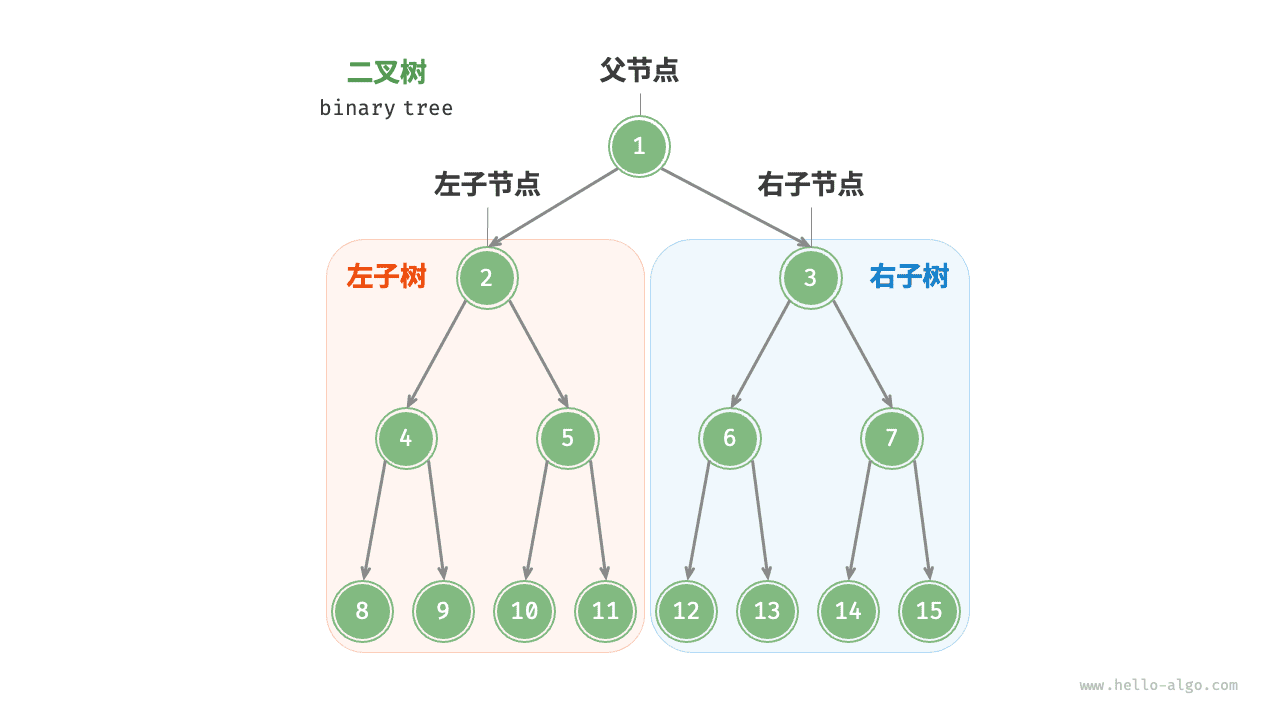Fig. 父结点、子结点、子树

## 7.1.1.   二叉树常见术语¶

• 「根结点 Root Node」：二叉树最顶层的结点，其没有父结点；
• 「叶结点 Leaf Node」：没有子结点的结点，其两个指针都指向 $$\text{null}$$
• 结点所处「层 Level」：从顶至底依次增加，根结点所处层为 1 ；
• 结点「度 Degree」：结点的子结点数量。二叉树中，度的范围是 0, 1, 2 ；
• 「边 Edge」：连接两个结点的边，即结点指针；
• 二叉树「高度」：二叉树中根结点到最远叶结点走过边的数量；
• 结点「深度 Depth」 ：根结点到该结点走过边的数量；
• 结点「高度 Height」：最远叶结点到该结点走过边的数量；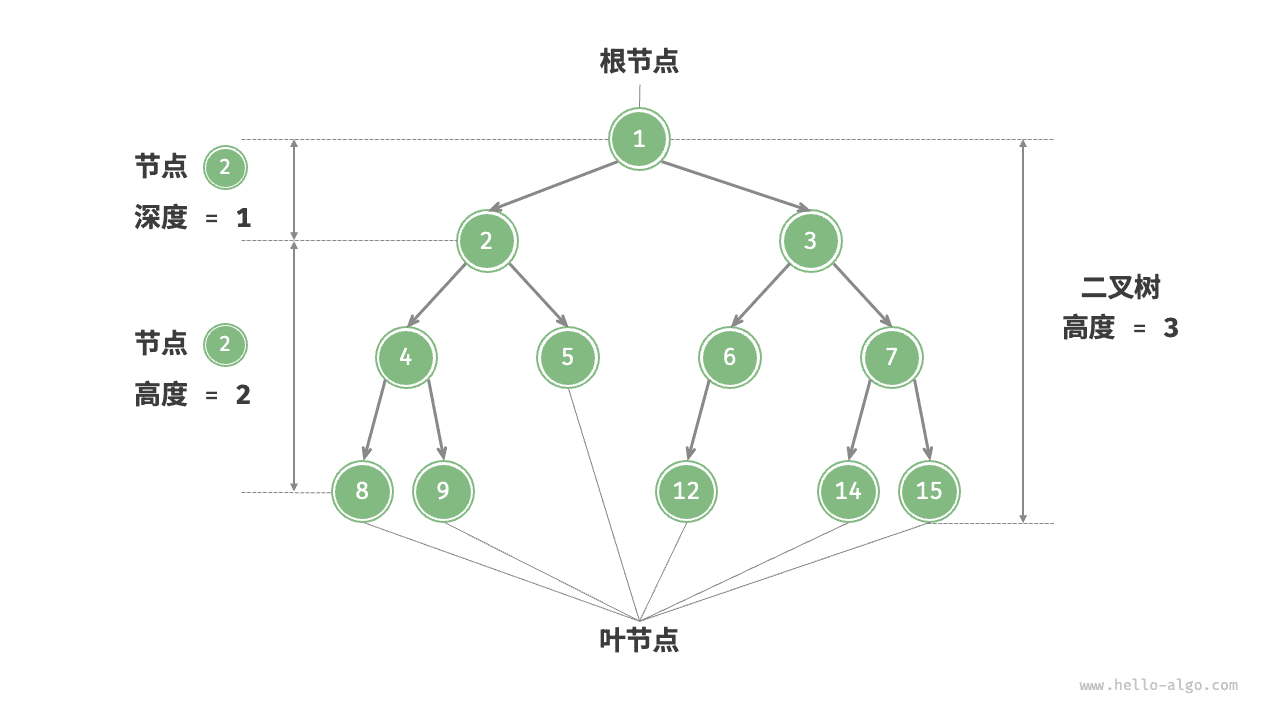Fig. 二叉树的常用术语

## 7.1.2.   二叉树基本操作¶

binary_tree.java
// 初始化结点
TreeNode n1 = new TreeNode(1);
TreeNode n2 = new TreeNode(2);
TreeNode n3 = new TreeNode(3);
TreeNode n4 = new TreeNode(4);
TreeNode n5 = new TreeNode(5);
// 构建引用指向（即指针）
n1.left = n2;
n1.right = n3;
n2.left = n4;
n2.right = n5;

binary_tree.cpp
/* 初始化二叉树 */
// 初始化结点
TreeNode* n1 = new TreeNode(1);
TreeNode* n2 = new TreeNode(2);
TreeNode* n3 = new TreeNode(3);
TreeNode* n4 = new TreeNode(4);
TreeNode* n5 = new TreeNode(5);
// 构建引用指向（即指针）
n1->left = n2;
n1->right = n3;
n2->left = n4;
n2->right = n5;

binary_tree.py
""" 初始化二叉树 """
# 初始化结点
n1 = TreeNode(val=1)
n2 = TreeNode(val=2)
n3 = TreeNode(val=3)
n4 = TreeNode(val=4)
n5 = TreeNode(val=5)
# 构建引用指向（即指针）
n1.left = n2
n1.right = n3
n2.left = n4
n2.right = n5

binary_tree.go
/* 初始化二叉树 */
// 初始化结点
n1 := NewTreeNode(1)
n2 := NewTreeNode(2)
n3 := NewTreeNode(3)
n4 := NewTreeNode(4)
n5 := NewTreeNode(5)
// 构建引用指向（即指针）
n1.Left = n2
n1.Right = n3
n2.Left = n4
n2.Right = n5

binary_tree.js
/* 初始化二叉树 */
// 初始化结点
let n1 = new TreeNode(1),
n2 = new TreeNode(2),
n3 = new TreeNode(3),
n4 = new TreeNode(4),
n5 = new TreeNode(5);
// 构建引用指向（即指针）
n1.left = n2;
n1.right = n3;
n2.left = n4;
n2.right = n5;

binary_tree.ts
/* 初始化二叉树 */
// 初始化结点
let n1 = new TreeNode(1),
n2 = new TreeNode(2),
n3 = new TreeNode(3),
n4 = new TreeNode(4),
n5 = new TreeNode(5);
// 构建引用指向（即指针）
n1.left = n2;
n1.right = n3;
n2.left = n4;
n2.right = n5;

binary_tree.c


binary_tree.cs
/* 初始化二叉树 */
// 初始化结点
TreeNode n1 = new TreeNode(1);
TreeNode n2 = new TreeNode(2);
TreeNode n3 = new TreeNode(3);
TreeNode n4 = new TreeNode(4);
TreeNode n5 = new TreeNode(5);
// 构建引用指向（即指针）
n1.left = n2;
n1.right = n3;
n2.left = n4;
n2.right = n5;

binary_tree.swift
// 初始化结点
let n1 = TreeNode(x: 1)
let n2 = TreeNode(x: 2)
let n3 = TreeNode(x: 3)
let n4 = TreeNode(x: 4)
let n5 = TreeNode(x: 5)
// 构建引用指向（即指针）
n1.left = n2
n1.right = n3
n2.left = n4
n2.right = n5

binary_tree.zig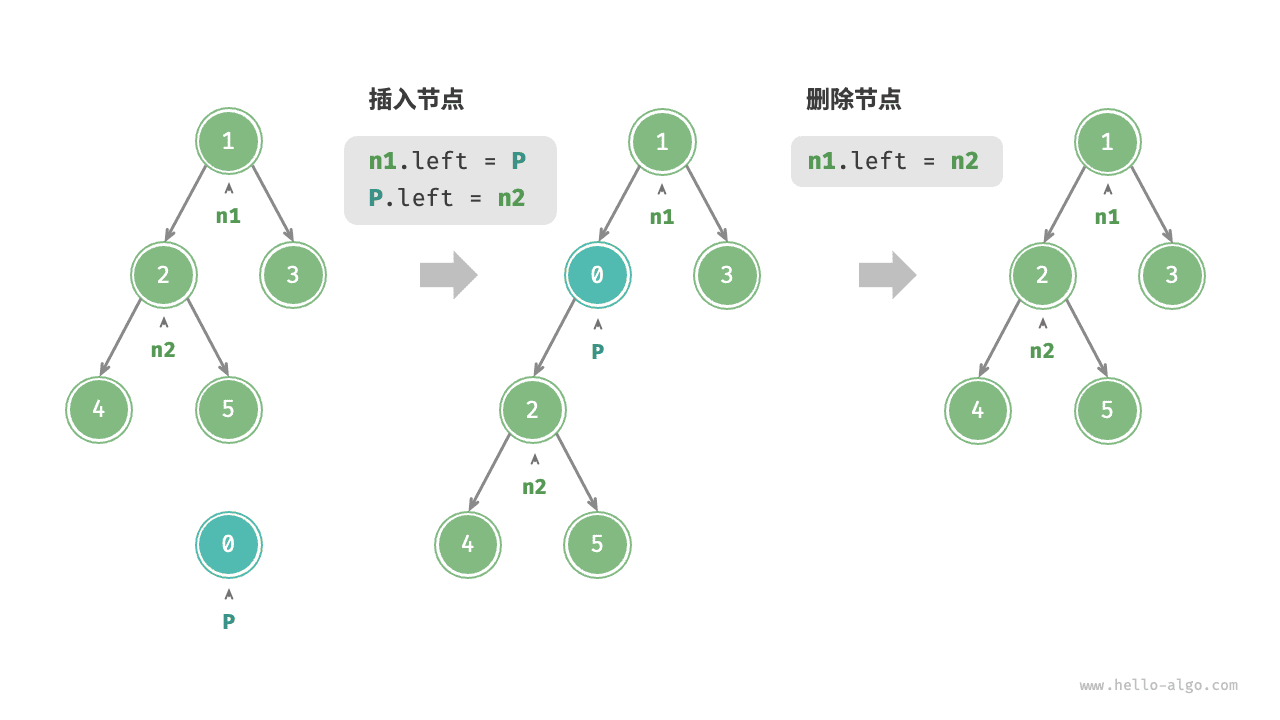Fig. 在二叉树中插入与删除结点

binary_tree.java
TreeNode P = new TreeNode(0);
// 在 n1 -> n2 中间插入结点 P
n1.left = P;
P.left = n2;
// 删除结点 P
n1.left = n2;

binary_tree.cpp
/* 插入与删除结点 */
TreeNode* P = new TreeNode(0);
// 在 n1 -> n2 中间插入结点 P
n1->left = P;
P->left = n2;
// 删除结点 P
n1->left = n2;

binary_tree.py
""" 插入与删除结点 """
p = TreeNode(0)
# 在 n1 -> n2 中间插入结点 P
n1.left = p
p.left = n2
# 删除结点 P
n1.left = n2

binary_tree.go
/* 插入与删除结点 */
// 在 n1 -> n2 中间插入结点 P
p := NewTreeNode(0)
n1.Left = p
p.Left = n2
// 删除结点 P
n1.Left = n2

binary_tree.js
/* 插入与删除结点 */
let P = new TreeNode(0);
// 在 n1 -> n2 中间插入结点 P
n1.left = P;
P.left = n2;
// 删除结点 P
n1.left = n2;

binary_tree.ts
/* 插入与删除结点 */
const P = new TreeNode(0);
// 在 n1 -> n2 中间插入结点 P
n1.left = P;
P.left = n2;
// 删除结点 P
n1.left = n2;

binary_tree.c


binary_tree.cs
/* 插入与删除结点 */
TreeNode P = new TreeNode(0);
// 在 n1 -> n2 中间插入结点 P
n1.left = P;
P.left = n2;
// 删除结点 P
n1.left = n2;

binary_tree.swift
let P = TreeNode(x: 0)
// 在 n1 -> n2 中间插入结点 P
n1.left = P
P.left = n2
// 删除结点 P
n1.left = n2

binary_tree.zig



Note

## 7.1.3.   常见二叉树类型¶

### 完美二叉树¶

「完美二叉树 Perfect Binary Tree」的所有层的结点都被完全填满。在完美二叉树中，所有结点的度 = 2 ；若树高度 $$= h$$ ，则结点总数 $$= 2^{h+1} - 1$$ ，呈标准的指数级关系，反映着自然界中常见的细胞分裂。

Tip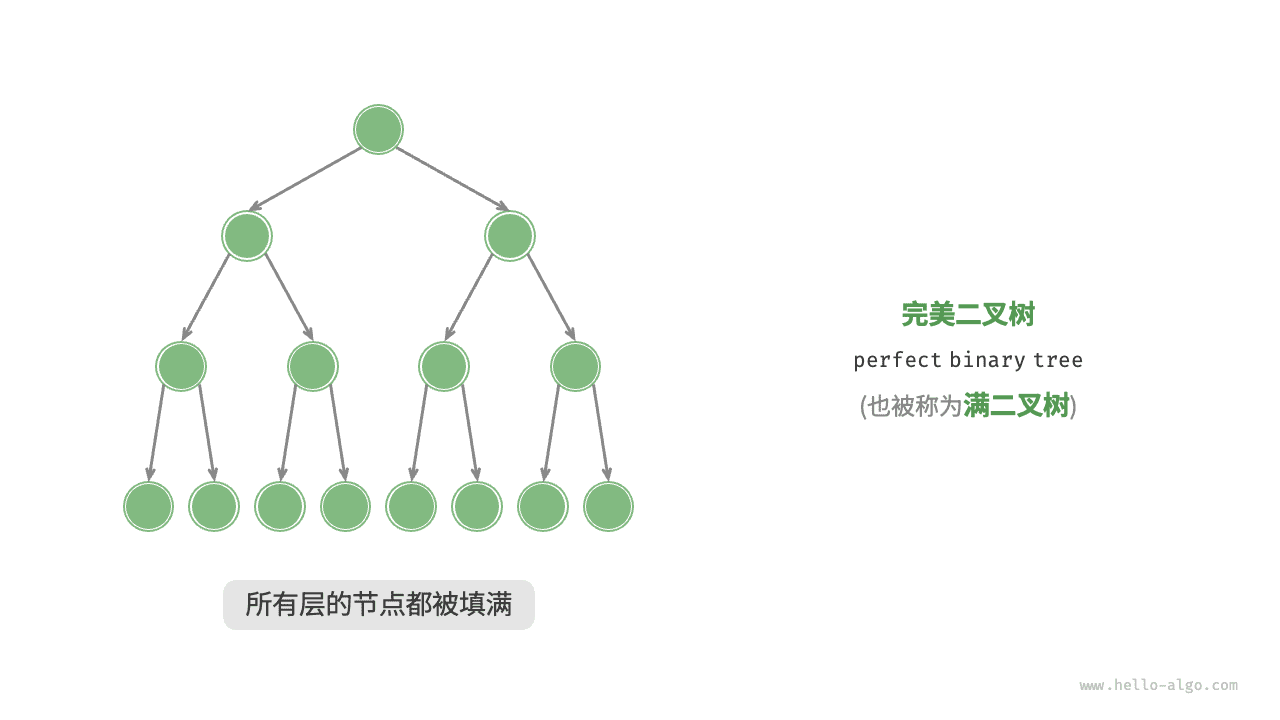Fig. 完美二叉树

### 完全二叉树¶

「完全二叉树 Complete Binary Tree」只有最底层的结点未被填满，且最底层结点尽量靠左填充。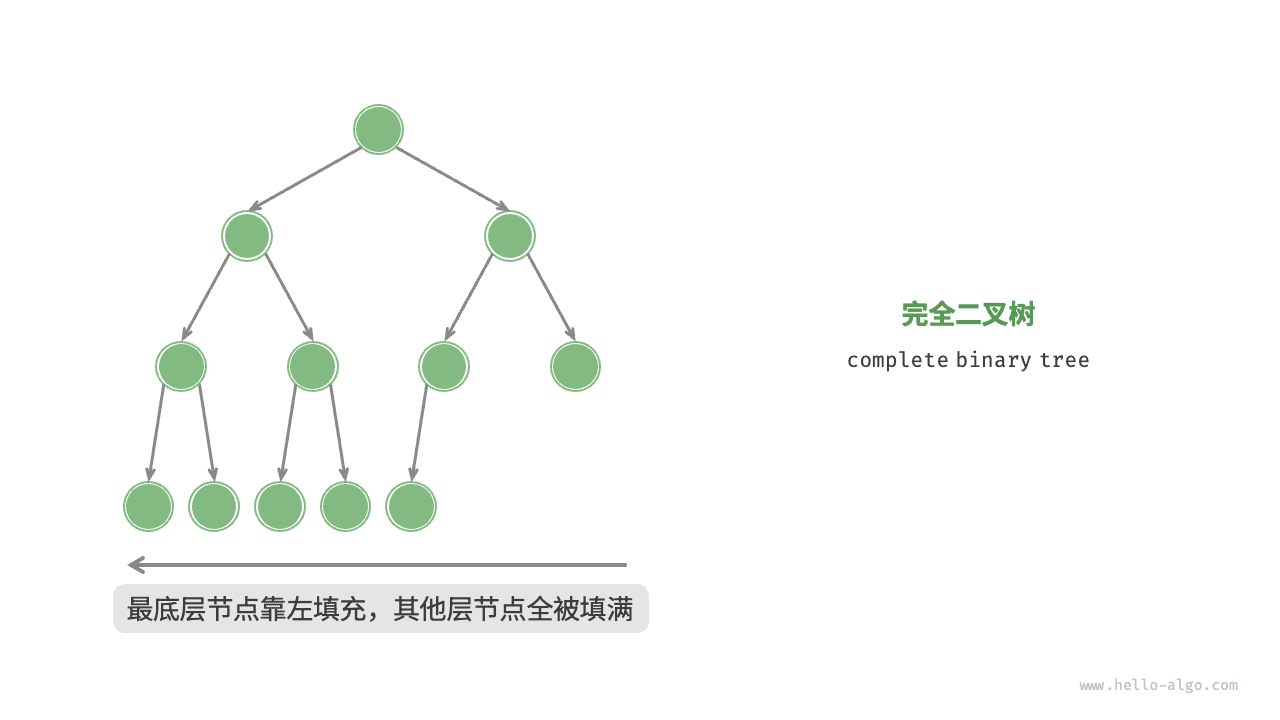Fig. 完全二叉树

### 完满二叉树¶

「完满二叉树 Full Binary Tree」除了叶结点之外，其余所有结点都有两个子结点。Fig. 完满二叉树

### 平衡二叉树¶

「平衡二叉树 Balanced Binary Tree」中任意结点的左子树和右子树的高度之差的绝对值 $$\leq 1$$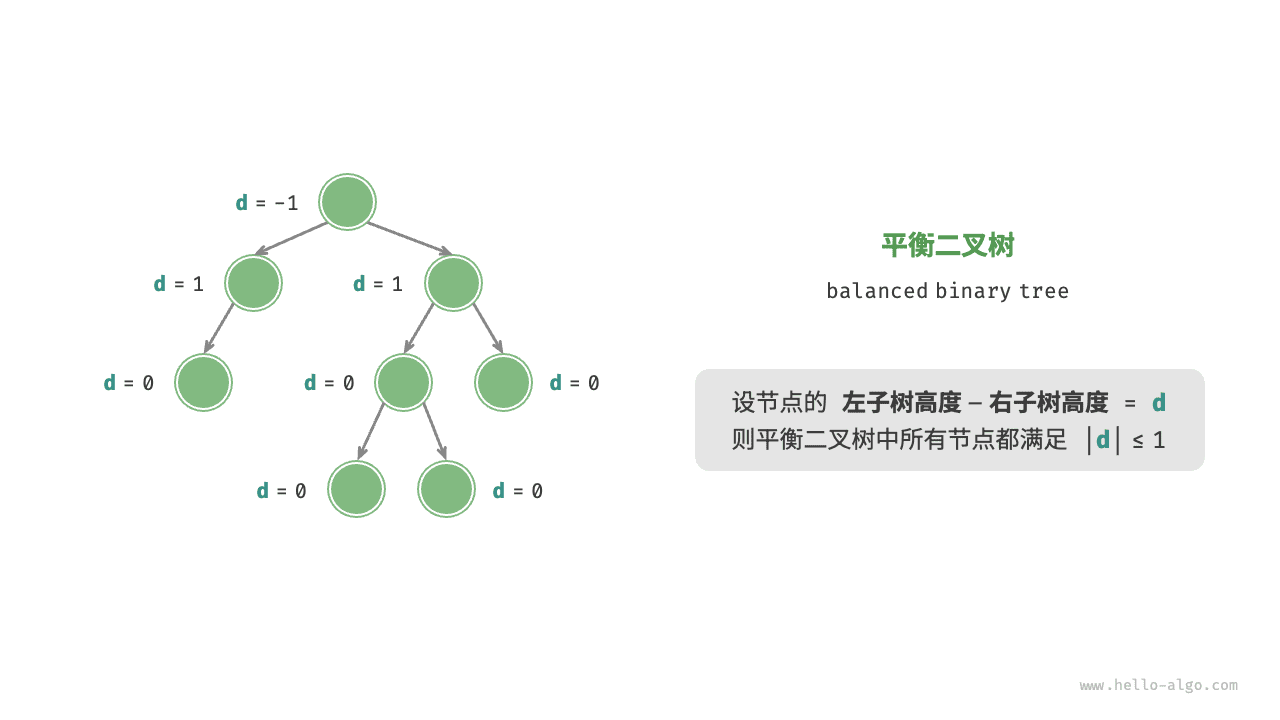Fig. 平衡二叉树

## 7.1.4.   二叉树的退化¶

• 完美二叉树是一个二叉树的“最佳状态”，可以完全发挥出二叉树“分治”的优势；
• 链表则是另一个极端，各项操作都变为线性操作，时间复杂度退化至 $$O(n)$$Fig. 二叉树的最佳与最二叉树的最佳和最差结构差情况

$$i$$ 层的结点数量 $$2^{i-1}$$ $$1$$

## 7.1.5.   二叉树表示方式 *¶Fig. 完美二叉树的数组表示Fig. 给定数组对应多种二叉树可能性

/* 二叉树的数组表示 */
// 使用 int 的包装类 Integer ，就可以使用 null 来标记空位
Integer[] tree = { 1, 2, 3, 4, null, 6, 7, 8, 9, null, null, 12, null, null, 15 };

/* 二叉树的数组表示 */
// 为了符合数据类型为 int ，使用 int 最大值标记空位
// 该方法的使用前提是没有结点的值 = INT_MAX
vector<int> tree = { 1, 2, 3, 4, INT_MAX, 6, 7, 8, 9, INT_MAX, INT_MAX, 12, INT_MAX, INT_MAX, 15 };

""" 二叉树的数组表示 """
# 直接使用 None 来表示空位
tree = [1, 2, 3, 4, None, 6, 7, 8, 9, None, None, 12, None, None, 15]

/* 二叉树的数组表示 */
// 使用 any 类型的切片, 就可以使用 nil 来标记空位
tree := []any{1, 2, 3, 4, nil, 6, 7, 8, 9, nil, nil, 12, nil, nil, 15}

/* 二叉树的数组表示 */
// 直接使用 null 来表示空位
let tree = [1, 2, 3, 4, null, 6, 7, 8, 9, null, null, 12, null, null, 15];

/* 二叉树的数组表示 */
// 直接使用 null 来表示空位
let tree: (number | null)[] = [1, 2, 3, 4, null, 6, 7, 8, 9, null, null, 12, null, null, 15];



/* 二叉树的数组表示 */
// 使用 int? 可空类型 ，就可以使用 null 来标记空位
int?[] tree = { 1, 2, 3, 4, null, 6, 7, 8, 9, null, null, 12, null, null, 15 };

/* 二叉树的数组表示 */
// 使用 Int? 可空类型 ，就可以使用 nil 来标记空位
let tree: [Int?] = [1, 2, 3, 4, nil, 6, 7, 8, 9, nil, nil, 12, nil, nil, 15]Fig. 任意类型二叉树的数组表示Fig. 完全二叉树的数组表示